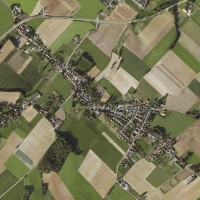Welcome to Bingelder.nl Photography - ConcertsHome   - Home Mardi Gras Indians   - MGI Calendar Photography Hobby Links Recent changes Contact C o n c e r t p h o t o g r a p h y C o n c e r t p h o t o g r a p h y C o n c e r t p h o t o g r a p h y C o n c e r t p h o t o g r a p h y C o n c e r t p h o t o g r a p h y C o n c e r t p h o t o g r a p h y C o n c e r t p h o t o g r a p h y C o n c e r t p h o t o g r a p h y C o n c e r t p h o t o g r a p h y C o n c e r t p h o t o g r a p h y C o n c e r t p h o t o g r a p h y C o n c e r t p h o t o g r a p h yThis site created with KompoZer with additional HTML-editing in Kate